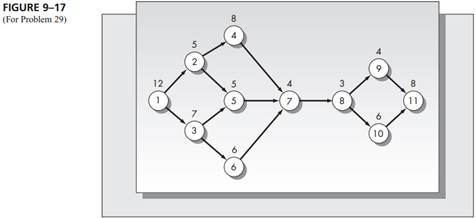Create an Account

Home / Questions / Consider the assembly line balancing problem represented by the network in Figure 9 17 The...

Consider the assembly line balancing problem represented by the network in Figure 9 17 The performance times are shown above the nodes a Determine a balance for

Consider the assembly line balancing problem represented by the network in Figure 9–17. The performance times are shown above the nodes.

a. Determine a balance for C =­ 15.

b. Determine a balance for C ­= 20.Jul 28 2020 View more View LessSubscribe To Get Solution